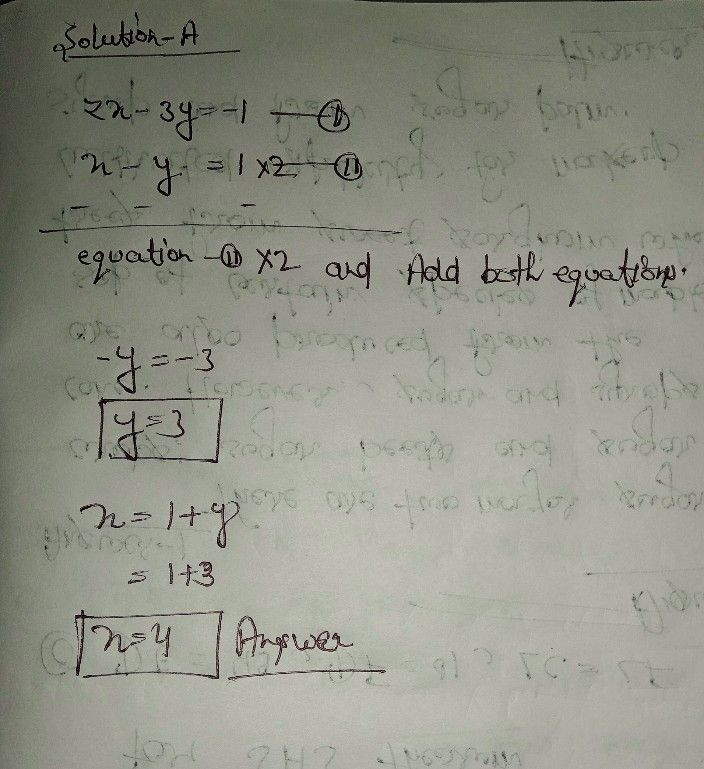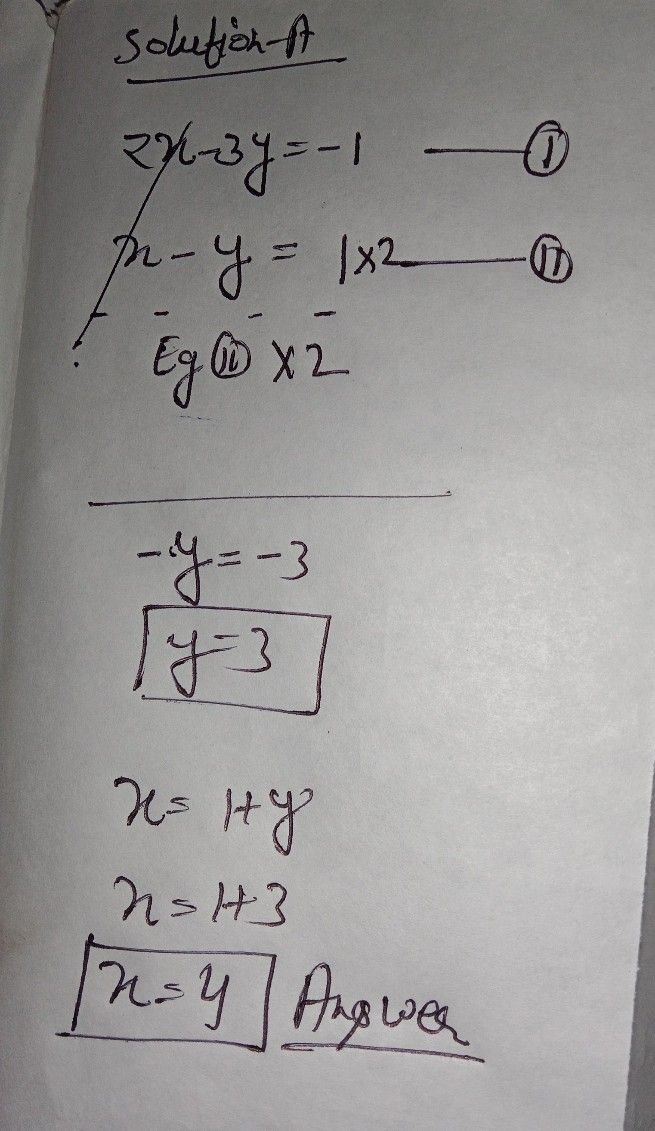Symbol
ProblemLearning Task $2.$ $A.$ Solve the systems of equations by $6$ graphing b) $\right)$ elimination c) substitution Eq. $1:$ $2x-3y=-1$ $Eq.2:$ $y=x-1$ B. Solve the problem using any method. The sum of two numbers is $32$ and the difference is $2$ Find the numbers
7th-9th grade
Other
SolutionQanda teacher - henryseeStudent
I don't understand the total answer
re-write it again please....I badly need it nowQanda teacher - henrysee
sorry for
waitStudent
ok I'll waitQanda teacher - henryseeStudent
thank you so much teachr?Qanda teacher - henrysee
tx